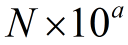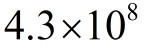# You have an original cell density of 4.3 x 10^8 CFU/mL. What is this number in its non-scientific notation or "regular" format?

Question
67 views

You have an original cell density of 4.3 x 10^8 CFU/mL. What is this number in its non-scientific notation or "regular" format?

check_circle

Step 1

Scientific notation is used in a standard way of writing very large and very small numbers so that they are easier to both compare and use in computations. To write in scientific notation, follow the formWhere N is a number between 1 and 10, but not 10 itself, and a is an integer (positive or negative number).

Step 2

In the given question colony forming unit (CFU) is used to determine the number of bacteria in the sample. So in this question number of bacteria isCFU/mL

That is actually 430000000 bacteria in 1 ml.

1) Move the decimal place to the left to create a new number from 1 up to 10. So N is 4.3.

2) Now determine the exponent, which is the number of times you moved the decimal from 430000000 to 4.3. In this, you moved the decimal 8 times; also, because you moved the decimal to the left, the exponent is positive. Therefore, a = 8, and...

### Want to see the full answer?

See Solution

#### Want to see this answer and more?

Solutions are written by subject experts who are available 24/7. Questions are typically answered within 1 hour.*

See Solution
*Response times may vary by subject and question.
Tagged in